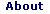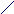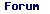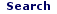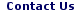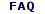Fri - 29 Sep 2023

WELCOME TO RFCABLES.ORG

Transmission-line analysis for a co-axial system

## Transmission line analysis for a coaxial system

### 1. Brief Introduction:

In this article, a brief analysis of the coaxial cable system is presented based on the electrical equivalent circuit of a transmission line. The aim here is to present some of the electrical and physical properties specific to the coaxial system.

### 2. Equivalent Circuit Model of a Transmission Line:

We start of by defining a transmission line using distributed circuit analysis. As this is well researched and well documented subject, only a brief extract of this analysis is presented here.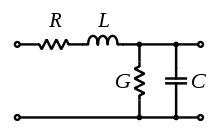Fig. Equivalent circuit model of a transmission line

Theoretically, in the distributed circuit element model, a transmission line is defined as an infinite periodic structure consisting of series and parallel combination of resistor (R), inductance (L), conductance (G) and capacitance (C) which when terminated into its characteristic impedance (Z0), results in maximum energy transfer between the source (VS, ZS) and the load (ZL) leading to a very desirable feature of transmission line called the matched condition. The lossy transmission line is represented by an equivalent circuit model wherein the energy storage in the magnetic field is done by the series inductance L per unit length; the energy storage in the electric field is done by the distributed shunt capacitance C per unit length; the power loss due to the conductors is represented by a series resistance R per unit length; and that due to the dielectric is included in the shunt conductance G per unit length.

In summary, some of the physical and electrical parameters determining a coaxial system are presented here.

### 3. Physical Parameters:

The physical parameters relate to the dimension (outer and inner conductor dimensions) of the coaxial cable and the material used in its construction (insulating dielectric material, center and outer conductor material)Fig. Cross-sectional view of a coaxial cable

• The outside diameter of the inner or center conductor, d.
• Inside diameter of the shield, D.
• Dielectric constant of the insulator, ε. In practice, relative dielectric constant εr, defined as: ε / ε0 is used, where ε0 is the free space dielectric constant. εeff is called the effective dielectric constant, and used in practice when the dielectric medium or the insulator is a mixture of different dielectric materials such as Polyethylene foam made from polyethylene and air.
• Magnetic permeability of the insulator, μ. However, in practice, relative permeability μr is used which is defined with respect to the permeability of free space, μ0 as: μr = μ/μ0. The relative permeability is approximately 1 for all practical purpose.

### 4. Fundamental Electrical Parameters:

As mentioned earlier the distributed circuit model analysis gives series and shunt electrical elements as listed below.

• Shunt capacitance per unit length, in farads per meter.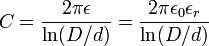• Series inductance per unit length, in henrys per meter.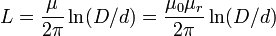• Series resistance per unit length, in ohms per meter. This parameter is the resistance of the inner conductor and the shield. Another factor, which affects this parameter, is the skin effect, wherein the propagating microwave signal tends to confine itself on the top layer or the 'skin' of the conductor, thus increasing the effective resistance. This phenomenon is prominent at higher microwave frequencies.
• Shunt conductance per unit length, in siemens per meter. The shunt conductance happens due to the dielectric loss of the insulator used. The measure of a dielectric material is called the loss tangent which shows how much lossy the material is. An insulating material with good dielectric properties will have a low loss tangent and consequently a low shunt conductance.

### 5. Derived electrical parameters:

The derived electrical parameters have dependencies on the fundamental electrical parameters like the L, C and R. These are listed below.

• Characteristic impedance in ohms (Ω). For a loss less coaxial cable (or for a practical coaxial cable having very low resistive loss), it is defined as the square root of the ratio of the inductance L per unit length to the capacitance C per unit length.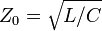From theory, it can be shown that Z0 can be determined in terms of the dimensions of the inner and outer conductors as the ratio of the inner (d) and outer (D) diameters and the dielectric constant (ε). It is given by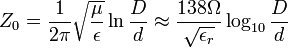It can be seen from the above equation that the characteristic impedance is frequency independent.
• Attenuation (loss) per unit length, in decibels per meter. This is an important parameter in applications where long runs of coaxial cable are required such as CATV distribution network, coaxial Ethernet etc. The contributing factors to attenuation, largely comes from the dielectric material filled inside the cable and the resistive losses associated with the inner conductor and the outer shield. Unlike the characteristic impedance parameter, attenuation depends on the frequency of the propagating microwave signal - higher the frequency; higher are the losses (or attenuation of the signal). As the frequency increases, a phenomenon called the 'skin effect' sets in. The skin effect results in the confinement of the microwave signal on the top surface of the inner conductor, thus reducing the effective diameter (and hence its effective resistance) of the inner conductor. The skin effect can be countered by increasing the diameter of the inner conductor, for example, skin effect resistance is halved if the cable's inner conductor diameter is doubled, thus reducing the skin losses at higher frequencies. If all other parameters remain the same (such as the dielectric loss, connector loss etc), this larger cable (with its inner conductor diameter doubled) will exhibit half the attenuation compared to the smaller cable (where the inner conductor diameter is not doubled), measured in terms of dB/meter. Cable attenuation can be mathematically expressed as,

Cable attenuation = conductor losses + dielectric losses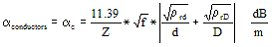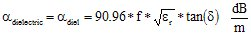ρr = 1 for copper, 10 for steel

Where,

d = Outside diameter of inner conductor in mm
D = Inside diameter of outer conductor in mm
εr = Relative dielectric constant
f = Frequency in GHz
ρrd = Inner conductor material resistivity relative to copper
ρrD = Outer conductor material resistivity relative to copper
δ = Skin depth
tan(δ) = loss tangent

• Velocity of propagation, in meters per second. Microwave energy is a form of electromagnetic energy, and when it propagates in air, it has the velocity of light. But as it propagates through the transmission media, it travels at a velocity which less than the velocity of light by a factor which is equal to the one over square root of εr and μr of the dielectric medium. The dielectric medium impedes the propagation of the microwave energy through the coaxial cable, leading to the reduction in its velocity. Mathematically, the velocity of propagation can be written as :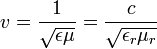It depends on the dielectric constant and the permeability (which is usually 1) of the dielectric material.

• Group delay: There is a finite time taken by a signal (of a particular frequency) to propagate through a coaxial cable, which constitutes the delay at that frequency. If the transit time through the cable remains constant at all frequencies, the phase is said to be linear w.r.t frequency. On the contrary, if the transit time is different at different frequency, it results in delay shift or non-linear phase shift. The non-linear phase shift w.r.t frequency tends to distort the propagating signal and is an undesirable feature for a transmission medium like a coaxial cable. Since the time delay experienced by the signal is different at different group of frequencies, the term group delay is introduced for delay experienced by a signal at different frequencies. The time delay of a coaxial line can be derived from the cable electrical parameters, L and C.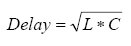Hence, by measuring the values of L and C we can compute the value of delay. For example, for a foot long RG58U cable, the measured capacitance is say 31.2 pF and inductance is, 76.8 nH. Using the above formula, we can compute time delay to be 1.55 ns. For an ideal transmission line, like the coaxial cable, the time delay increases linearly with the length and the characteristic impedance remains constant. As the signal travels from one end of the cable to the other, its phase shifts (i.e. the difference of phase at the input to the phase at its output) linearly with the frequency as expressed by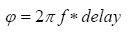where phase Φ is in radians and frequency in Hertz. Converting the above, into degrees, yields the following expression,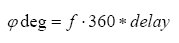A vector network analyzer or VNA is used to measure delay by measuring the phase change over a change of frequency.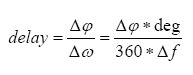The definition of group delay as the negative derivative of the phase, Φin degrees with respect to frequency f is expresses as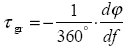For practical reasons, the VNA measures a difference quotient rather than a differential quotient, which yields a good approximation to the group delay, provided the variation of phase is not too non-linear in the observed frequency range Δf, called the aperture.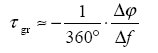The figure below illustrates ΔΦ = Φ2 – Φ1 and  Δf = f2 – f1 for linearly decreasing phase response, such as that of a delay line.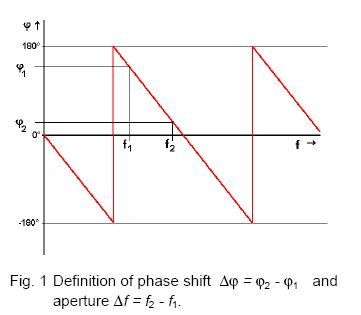The aperture  Δf should be chosen in accordance with the desired measurement accuracy and the variation of the group delay of the DUT versus frequency.

• Cutoff frequency is the highest frequency up to which single mode of propagation is possible inside a coaxial system, such as the dominant TEM mode. It is determined to be the frequency beyond which other modes of excitation or wave propagation occur. The cutoff frequency is dependent on the cable dimensions (outer and inner conductor) and the dielectric material properties (εr and μr). For example, for determining the cutoff frequency for a TE01 mode of propagation, the average circumference of the insulator isπ(D + d) / 2 is taken into account. For a length of dielectric medium equal to one wavelength of the propagating microwave signal, the cutoff frequency for TE01 mode is given by: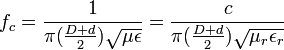Using the values of constants such as velocity of light, c and expressing the result in SI and English unit systems can further simplify the above equation. Assuming R to be equal to 1 for the dielectric material used and expressing frequency in GHz (instead of Hz) we arrive at the following simplified equations.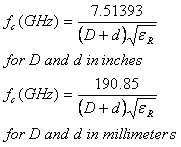• Peak Voltage determines the capability of handling peak energy voltage swings in the microwave signal propagating through the coaxial cable. From the literature it can be shown that it depends on the physical dimension of the cable and can be expresses as: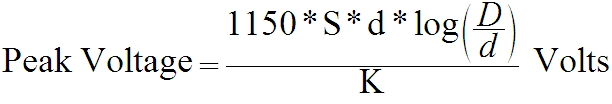Where, S = maximum voltage gradient of cable insulation in volts/mm
K = a safety factor

 Name: Email: Subject: Comment: characters left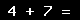Back

## Anand Software and Traini...Contact DetailsCompany Profile

More From Anand Software and Training Private Limited

## ArticlesRF and Microwave Connectors - Part 1RF and Microwave Connectors-Part 2RF and Microwave Connectors Part-3Co-axial Cables - An IntroductionTransmission-line analysis for a co-axial systemVideo CablesAudio Cables and ConnectorsMicrowave Transmission (Telecom (Wireless and Antenna Applications), Military, Test & Measurement, and Aerospace)Coaxial RF Cables Classification GuideCoaxial Cable Selection GuideRF Cable Assembly Equipment & ToolsRF Cable TestingCellular Phones - A Technology ReviewNoise Figure Measurements